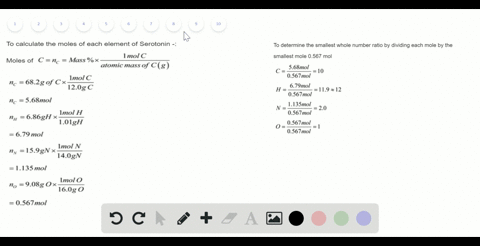Sign up for our free STEM online summer camps starting June 1st!View Summer Courses### Serotonin is a compound that conducts nerve impul…

01:52Auburn University Main Campus

Need more help? Fill out this quick form to get professional live tutoring.

Get live tutoring
Problem 92

(a) One molecule of the antibiotic penicillin G has a mass of $5.342 \times 10^{-21} \mathrm{g} .$ What is the molar mass of penicillin G? (b) Hemoglobin, the oxygen-carrying protein in red blood cells, has four iron atoms per molecule and contains 0.340$\%$ iron by mass. Calculate the molar mass of hemoglobin.

(a) 3216 $\mathrm{g} / \mathrm{mol}$
(b) 65700 $\mathrm{g} / \mathrm{mol}$

## Discussion

You must be signed in to discuss.

## Video Transcript

So in first part of this problem, we have given way the mass ofthe one molecule off and then barbarity, Penicillin, G. And we have to find out the molar mass of this and a magic. So as we know that the molar mass is the the mass off, one more ofthis substance. And since one more one more nickel off the substance condense this much mass and we know that one more of the substances contained now six point zero two times ten to the world twenty three molecules. So if they're if this is the mass ofthe one molecule, that means one mall of this antibiotic R will contain these times six point or dog germs. You know the word pointed cream gram of mass. So the molar mass off the penicillin G? No. It will be equal to the mass ofthe one molecule, which is five burned three for two times ten to the poor, minus two into one gram times the number of molecules in one matloff penicillin G, which is six point or two times ten to the part twenty three molecules. And this'LL give us a valve off. Um three two one five point a date from our mall. So this is the molar mass off the centre party. Hey. And then in second part of this problem we're given Ah, the person does off iron atoms, which is contained in hemoglobin on were said that in hemoglobin, in one molecule of him, a blooming contains four iron atoms and we're given on the mass percent is off Iran. We have to calculate the molar mass of hemoglobin. So we have a formula which relates the person mass off. Ah, often element in a substance with the molar mass of the substance, which is like this. So here you can see that the person must composition often element. They're called to the number of phantoms of the element times that'LL make weight of development over the formal await or the Mueller mass off them. Um, substance. So you're trying to find out this molar mass, so we know them. Ah, mass percentages of irony. We know the number off Adam's off iron in which is present and hemoglobin and we can find out the atomic weight off day element. And from all this data, we can find out the molar mass of hemoglobin. So if we just there Is this the data we have to the person mas we're given as zero point three four zero percent equal toe? You know, the number off Adam's off Iran present is given us for in hemoglobin. And the adequate off irony is fifty five point and wait for me, and we have to find out the molar mass off hemoglobin. So if we just, um, realities, we confined the molar mass off hemoglobin, which will be equal to four times fifty five point eight for Graham. Um, over the mass percentages point three four. And his persons is this person, we have to multiply the whole as hundreds, and this will give us a value off. Six, five, six, nine, four times one toe. Crump are more. So this is the Moler mass off hemoglobin, which is six five, six nine four or sixty five thousand six hundred ninety four one. Twelve Grandpa mall are from a glowing